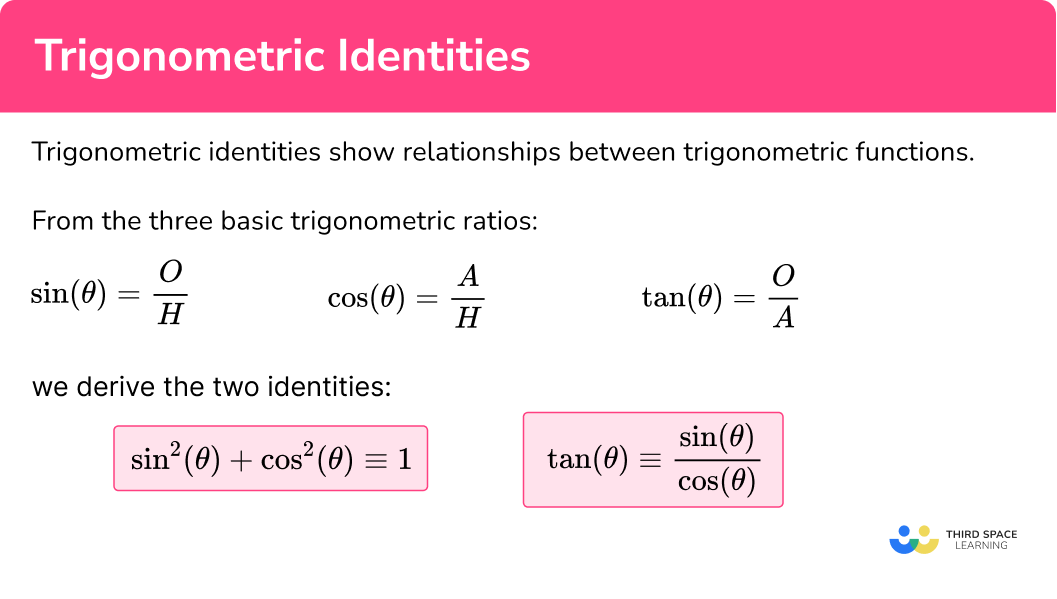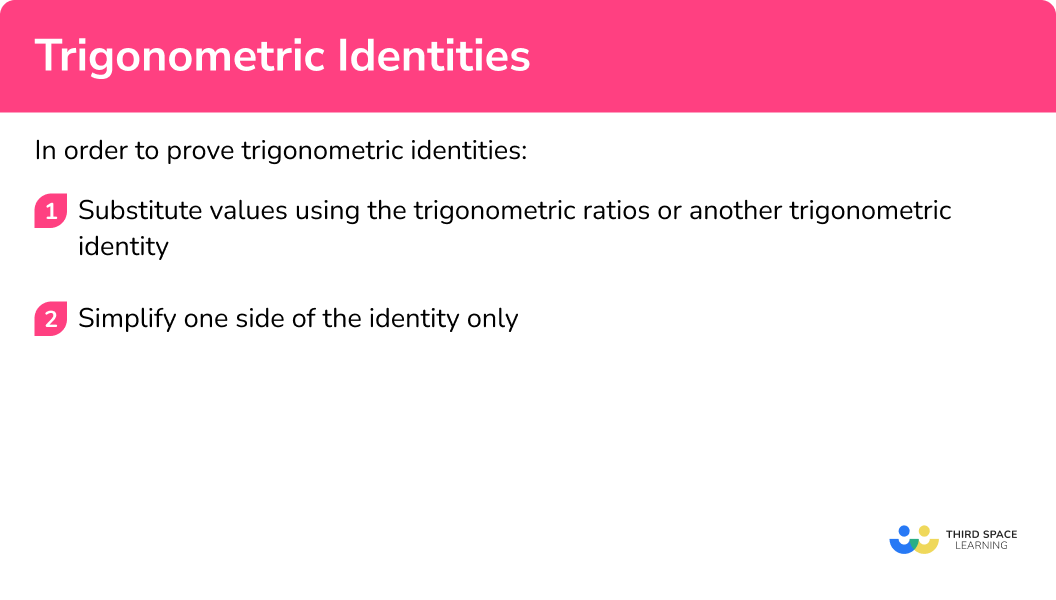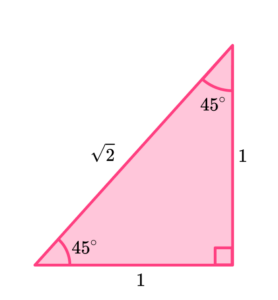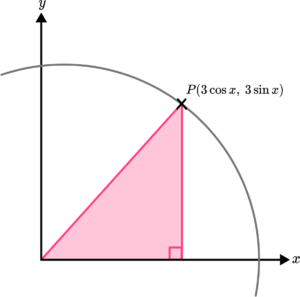# Trig Identities

Here we will learn about trigonometric identities, including recognising and working with key trigonometric identities.

There are also trigonometry worksheets based on Edexcel, AQA and OCR exam questions, along with further guidance on where to go next if you’re still stuck.

## What are trigonometric identities?

Trigonometric identities show relationships between trigonometric functions. These are not examined in GCSE Mathematics but are covered in Level 2 Further Mathematics, Additional Mathematics and IGCSE Further Mathematics courses.

An identity is an equation that is always true.

For example, when we expand the bracket 2(x+1) we get 2x+2. These two functions of x are identical and so we use the identity symbol (a triple equals symbol) to show that 2(x+1) \equiv 2x+2.

We can do the same with the trigonometric ratios of sine, cosine, and tangent.

• \sin(\theta)=\frac{O}{H}
• \cos(\theta)=\frac{A}{H}
• \tan(\theta)=\frac{O}{A}

Where O = Opposite, A = Adjacent, and H = Hypotenuse for the right-angled triangle with angle \theta (theta).

Step-by-step guide: SOHCAHTOA

There are two trigonometric identities that we will look at. These are,

\tan(\theta)\equiv\frac{\sin(\theta)}{\cos(\theta)} \ and \ \sin^2(\theta)+\cos^2(\theta)\equiv1.

Here \sin^2(\theta) is another way of writing (\sin(\theta))^2 .

These two identities will help us prove further identities, using the trigonometric ratios when necessary.

### What are trigonometric identities?## How to prove trigonometric identities

In order to prove trigonometric identities:

1. Substitute values using the trigonometric ratios or another trigonometric identity.
2. Simplify one side of the identity only.

### Explain how to prove trigonometric identities## Trigonometric identities examples

### Example 1: the relationship between sin, cos and tan

Prove that \ \frac{\sin(\theta)}{\cos(\theta)} \equiv \tan(\theta).

1. Substitute values using the trigonometric ratios or another trigonometric identity.

We need to show that \tan (\theta) is the same as \sin (\theta) \div \cos (\theta). To do this we can use the trigonometric ratios.

We know that \ \sin(\theta)=\frac{O}{H}, \ \cos(\theta)=\frac{A}{H}, \ and \ \tan(\theta)=\frac{O}{A}.

As we need to divide \sin(\theta) by \cos(\theta) , this is the same as dividing \frac{O}{H} by \frac{A}{H}.

So we have,

\sin(\theta) \div \cos(\theta) = \frac{O}{H} \div \frac{A}{H}.

2Simplify one side of the identity only.

Simplifying the right hand side, we have

\begin{aligned} &\frac{O}{H}\div\frac{A}{H} \\\\ = & \, \frac{O}{H}\times\frac{H}{A} \\\\ =& \, \frac{OH}{AH} \\\\ =& \, \frac{O}{A} \\\\ =& \, \tan(\theta) \end{aligned}

Therefore \ \frac{\sin(\theta)}{\cos(\theta)} \equiv \tan(\theta).

### Example 2: using Pythagoras’ theorem to prove a trigonometric identity

Prove that \ \sin^2(\theta)+\cos^2(\theta)\equiv1.

Substitute values using the trigonometric ratios or another trigonometric identity.

Simplify one side of the identity only.

### Example 3: proving identities using trig ratios

Prove that \sin(\theta)\div\tan(\theta)\equiv\cos(\theta).

Substitute values using the trigonometric ratios or another trigonometric identity.

Simplify one side of the identity only.

### Example 4: proving identities using exact trig values

Prove that \cos(60)=1-\sin(30) using the right-angled triangle below.

Substitute values using the trigonometric ratios or another trigonometric identity.

Simplify one side of the identity only.

### Example 5: proving identities featuring all three trig ratios

Prove that \cos(\theta)\times\tan(\theta)\times\sin(\theta)\equiv\sin^{2}(\theta).

Substitute values using the trigonometric ratios or another trigonometric identity.

Simplify one side of the identity only.

### Example 6: using the trigonometric identity sin2(θ) + cos2(θ) = 1

Prove that 6(3\sin^{2}(\theta))+2(9\cos^{2}(\theta))=18.

Substitute values using the trigonometric ratios or another trigonometric identity.

Simplify one side of the identity only.

### Common misconceptions

• Treating an identity as an equation that needs balancing

An identity cannot be balanced. It is a statement that two expressions or functions are mathematically identical. For example, 3x+6\equiv{3}(x+2) as when we factorise 3(x+6) we get the expression 3(x+2).

• Mixing up SOHCAHTOA

It is key to remember the three trig ratios that are written as the mnemonic SOHCAHTOA. This way you will not confuse the relationships between the sides and the angles.

### Practice trigonometric identities questions

1. Which of the following trigonometric identities is mathematically correct?

\sin(\theta)\times\cos(\theta)=\tan(\theta)\sin(\theta)+\cos(\theta)=\tan(\theta)\sin(\theta)\div\cos(\theta)=\tan(\theta)\sin(\theta)-\cos(\theta)=\tan(\theta)As \sin(\theta)=\frac{O}{H} and \cos(\theta)=\frac{A}{H},

\sin(\theta)\div\cos(\theta)=\frac{O}{H}\div\frac{A}{H}=\frac{O}{H}\times\frac{H}{A}=\frac{OH}{HA}=\frac{O}{A}=\tan(\theta).

2. Which of the following trigonometric identities is mathematically correct?

\sin^{2}(\theta)-\cos^{2}(\theta)=1\sin^{2}(\theta)+\cos^{2}(\theta)=1\sin(\theta)-\cos(\theta)=1\sin(\theta)+\cos(\theta)=1As \sin(\theta)=\frac{O}{H}, multiplying both sides by H to make O the subject,
we have O=H\sin(\theta).

As \cos(\theta)=\frac{A}{H}, multiplying both sides by H to make A the subject,
we have A=H\cos(\theta).

As the base is H\cos(\theta), the height is H\sin(\theta), and the hypotenuse is H, we can substitute these into Pythagoras’ theorem to get,

(H\sin(\theta))^{2}+(H\cos(\theta))^{2}=H^{2}

\begin{aligned} &(H\sin(\theta))^{2}+(H\cos(\theta))^{2}\\\\ =&H^{2}\sin^{2}(\theta)+H^{2}\cos^{2}(\theta)\\\\ =&H^{2}(\sin^{2}(\theta)+\cos^{2}(\theta)) \end{aligned}

This means that as H^{2}(\sin^{2}(\theta)+\cos^{2}(\theta))=H^{2}, \ \sin^{2}(\theta)+\cos^{2}(\theta)=1 as H^{2}\times{1}=H^{2}.

3. Which of the following trigonometric functions is identical to \frac{\tan(\theta)}{\sin(\theta)}?

\cos(\theta)\sin^{-1}(\theta)\frac{1}{\cos(\theta)}\cos^{2}(\theta)As \sin(\theta)=\frac{O}{H}, \ \tan(\theta)=\frac{O}{A}, and \cos(\theta)=\frac{A}{H},

\tan(\theta)\div\sin(\theta) is equivalent to

\begin{aligned} &\frac{O}{A}\div\frac{O}{H}\\\\ =\; & \frac{O}{A}\times\frac{H}{O}\\\\ =\; & \frac{OH}{AO}\\\\ =\; & \frac{H}{A}\\\\ =\; & \frac{1}{\cos(\theta)} \end{aligned}

4. Which solution is identical to 2\cos(45)\times\sin(45)?\sqrt{2}12\sqrt{2}22\cos(45)\times\sin(45)=2\times\frac{1}{\sqrt{2}}\times\frac{1}{\sqrt{2}}=2\times\frac{1}{2}=1

5. Which solution is identical to \cos(\theta)\times\tan(\theta)\div\sin(\theta)?

1\tan^{2}(\theta)\frac{1}{\tan(\theta)}\frac{\cos^{2}(\theta)}{\sin^{2}(\theta)}\cos(\theta)\times\tan(\theta)\div\sin(\theta)=\cos(\theta)\times\frac{\sin(\theta)}{\cos(\theta)}\div\sin(\theta)=\frac{\cos(\theta)\sin(\theta)}{\cos(\theta)\sin(\theta)}=1

6. Which solution is identical to \frac{\sin(\theta)}{\sqrt{1-\sin^{2}(\theta)}}?

\sin(\theta)\cos(\theta)\tan(\theta)\cos^{2}(\theta)\frac{\sin(\theta)}{\sqrt{1-\sin^{2}(\theta)}}=\frac{\sin(\theta)}{\sqrt{\cos^{2}(\theta)}}=\frac{\sin(\theta)}{\cos(\theta)}=\tan(\theta)

### Trigonometric identities questions

1. Prove that \frac{\sqrt{1-\cos^{2}(\theta)}}{\cos(\theta)}\equiv \tan(\theta).

(2 marks)

\sqrt{1-\cos^{2}(\theta)} = \sin(\theta)

(1)

\frac{\sin(\theta)}{\cos(\theta)}= \tan(\theta)

(1)

2. (a) Expand and simplify (\sin x + \cos x)^{2}.

(b) Use your solution to part a) to prove that (\sin x + \cos x)^{2} + (\sin x – \cos x)^{2} \equiv 2.

(6 marks)

(a)

(\sin x + \cos x) (\sin x + \cos x) =

(1)

\sin^{2} x + 2 \cos x \sin x + \cos^{2} x

(1)

(b)

(\sin x-\cos x)^{2} = \sin^{2} x-2\cos x \sin x + cos^{2} x

(1)

\sin^{2}x+2\cos x \sin x+ \cos^{2}x+ \sin^{2} x-2 \cos x \sin x+\cos^{2}x=2\sin^{2}x+2\cos^{2}x

(1)

2\sin^{2}x+2 \cos^{2}x =2(\sin^{2}x+\cos^{2}x)

(1)

2\sin^{2}x+2 \cos^{2}x =2(1) =2

(1)

3. The axes below show a circle where the point P(H \cos x, \ H \sin x) is a point on the circumference of the circle.Prove that the radius of the circle is 3.

(4 marks)

(3 \cos x)^{2}+(3 \sin x)^{2}=r^{2}

(1)

9 \cos^{2} x + 9 \sin^{2} x =r^{2}

(1)

9 (\cos^{2} x + \sin^{2} x) = 9

(1)

r=\sqrt{9}=3

(1)

## Learning checklist

You have now learned how to:

• Use Pythagoras’ theorem, a^2+b^2=c^2 to prove the trigonometric ratios \sin(\theta)=\frac{O}{H}, \ \cos(\theta)=\frac{A}{H} and \tan(\theta)=\frac{O}{A}
• Use the trigonometric ratios to prove trigonometric identities
• Extend and formalise your knowledge of ratio and proportion, including trigonometric ratios

## More trigonometric functions and identities

Other trig functions that are studied in a level Mathematics include

• Secant (sec \theta)
• Cosecant (csc \theta)
• Cotangent (cot \theta)

We also study trigonometric values beyond 90 degrees (outside of the limits of a right-angled triangle). These include double-angle formulas (double-angle identities), and conversely half-angle formulas (half-angle identities). These allow us to form trigonometric equations.

At A-level, we use a different measurement for an angle called radians where 2\pi radians is a full turn (360^{\circ}), \pi radians is a half turn (180^{\circ}), \frac{\pi}{2} radians is a quarter turn (90^{\circ}), etc.

We also work with the integrals and derivatives of different trigonometric functions to form other trig equations.

## Still stuck?

Prepare your KS4 students for maths GCSEs success with Third Space Learning. Weekly online one to one GCSE maths revision lessons delivered by expert maths tutors.

Find out more about our GCSE maths tuition programme.